# Math in Focus Grade 3 Chapter 16 Practice 2 Answer Key Converting Hours and Minutes

This handy Math in Focus Grade 3 Workbook Answer Key Chapter 16 Practice 2 Converting Hours and Minutes provides detailed solutions for the textbook questions.

## Math in Focus Grade 3 Chapter 16 Practice 2 Answer Key Converting Hours and Minutes

Complete each number bond.

Example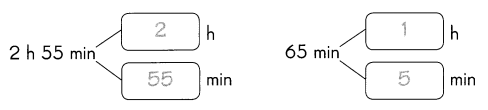Question 1.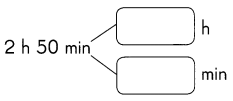Hour and minute: Hour and minutes are the units used to measure the time. One hour is equal to sixty minutes. But we can write minutes as a decimal number of hours. For example, half an hour (1/2 or 0.5 hours) cannot be written as 50 minutes. Half of an hour is equal to 30 minutes.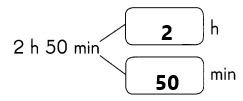1 hour=60 minute
1 minute=60 second

Question 2.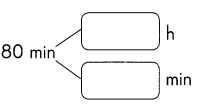Hour and minute: Hour and minutes are the units used to measure the time. One hour is equal to sixty minutes. But we can write minutes as a decimal number of hours. For example, half an hour (1/2 or 0.5 hours) cannot be written as 50 minutes. Half of an hour is equal to 30 minutes.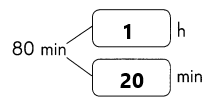This can be calculated as:
1 hour=60 minute, so I wrote 1 in hour box
If we add 20 to the 60 then we get:60+20=80.
so I add 20 in the minute’s box.
Therefore, 80 minutes is nothing but 1 hour 20 minutes.

Tell the time in minutes.

Example

In the afternoon, Tom takes 1 hour 15 minutes to do his homework. How many minutes are in 1 hour 15 minutes?1 h 15 min
= 60 min + 15 min
= 75 min
There are 75 minutes in 1 hour 15 minutes.

Tell the time in minutes.

Question 3.
Katie took 3 hours 20 minutes to sew two sets of curtains. How many minutes are in 3 hours 20 minutes?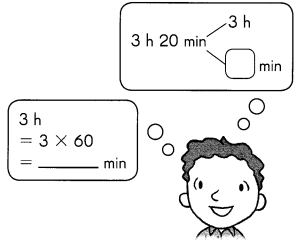3h 20 min
= _______ min+ ________ min
+ ______ min+ ______ min
= __________ min
There are __________ minutes in 3 hours 20 minutes.Explanation:
3 hour 20 minutes:
1 hour=60 minute
So multiply 3 by 60. Then we get 60×3=180.
Here, already 20 minutes is given and 3 hours also I converted into minutes that is 180.
Now add these two minutes that are 180 and 20.
=180 minutes+20 minutes
=200 minutes.

Express the time in minutes.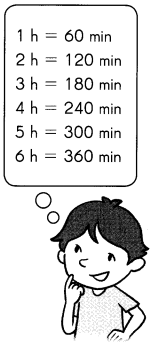Question 4.
1 h 20 min = ________ min + 20 min = ________ min
Explanation:
1 hour 20 minutes can be written in minutes as:
1 hour=60 minute’s. This is the basic thing we need to remember.
Here already given,20 minutes. I converted 1 hour to minutes that is 60.
Therefore, 1 h 20 min=80 minutes.

Question 5.
2 h 48 min = 120 min + _________ min = _________ min
Explanation:
1 hour=60 minutes
2hours=60×60=120 minutes. Already hours are converted in to minutes.
In the question 2 h 48 min=120+48=168 minutes.
Therefore, 2 hour 48 minute’s=168 minutes.

Question 6.
1 h 40 min = _______ min
Explanation:
1 hour 40 minutes can be written in minutes as:
1 hour=60 minute’s. This is the basic thing we need to remember.
Here already given,40 minutes. I converted 1 hour to minutes that is 60.
Therefore, 1 h 40 min=100 minutes.

Question 7.
2 h 25 min = _______ min
1 hour=60 minute’s. This is the basic thing we need to remember.
2 hour 25 minutes can be written in minutes as:
2 hour=60×60 minutes=120 min.
Here already given,25 minutes. I converted 2 hours to minutes that is 120.
Therefore, 2 h 25 min=145 minutes.

Question 8.
3 h 5 min = _______ min
1 hour=60 minute’s. This is the basic thing we need to remember.
3 hour 5 minutes can be written in minutes as:
3 hour=3×60 minutes=180 min.
Here already given, 5 minutes. I converted 3 hours to minutes that is 180.
Therefore, 3 h 5 min=185 minutes.

Question 9.
5 h 50 min = _______ min
1 hour=60 minute’s. This is the basic thing we need to remember.
5 hour 50 minutes can be written in minutes as:
5 hour=5×60 minutes=300 min.
Here already given, 50 minutes. I converted 5 hours to minutes that is 300.
Therefore, 5 h 50 min=350 minutes.

Question 10.
4 h 16 min = __________ min
1 hour=60 minute’s. This is the basic thing we need to remember.
4 hour 16 minutes can be written in minutes as:
4 hour=4×60 minutes=240 min.
Here already given, 16 minutes. I converted 4 hours to minutes that is 240.
Therefore, 4 h 16 min=256 minutes.

Question 11.
6 h 30 min = ___________ min
1 hour=60 minute’s. This is the basic thing we need to remember.
6 hour 30 minutes can be written in minutes as:
6 hour=6×60 minutes=360 min.
Here already given, 30 minutes. I converted 6 hours to minutes that is 360.
Therefore, 6 h 30 min=390 minutes.

Express the time in hours.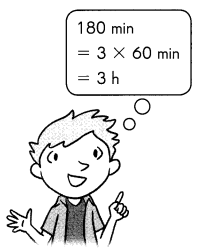Question 12.
180 min = __________ h
To convert minutes into hours, we have to divide the number of minutes by 60.
we already know, 1 hour=60 minute’s.
We need to convert 180 minutes into hours. Assume it as ‘X’ hours.
Now calculate hours. This can be done as:
180 minutes= X hours.
1 hour = 60 minute’s.
Divide 180 and 60 to get the hours.
X=180/60
X=3 hours.
Therefore, 180 minutes=3 hours.

Question 13.
360 min = ____________ h
To convert minutes into hours, we have to divide the number of minutes by 60.
we already know, 1 hour=60 minute’s.
We need to convert 360 minutes into hours. Assume it as ‘X’ hours.
Now calculate hours. This can be done as:
360 minutes= X hours.
1 hour = 60 minute’s.
Divide 360 and 60 to get the hours.
X=360/60
X=6 hours.
Therefore, 360 minutes=6 hours.

Question 14.
120 min = ____________ h
To convert minutes into hours, we have to divide the number of minutes by 60.
we already know, 1 hour=60 minute’s.
We need to convert 120 minutes into hours. Assume it as ‘X’ hours.
Now calculate hours. This can be done as:
120 minutes= X hours.
1 hour = 60 minute’s.
Divide 120 and 60 to get the hours.
X=120/60
X=2 hours.
Therefore, 120 minutes=2 hours.

Question 15.
240 min = ___________ h
To convert minutes into hours, we have to divide the number of minutes by 60.
we already know, 1 hour=60 minute’s.
We need to convert 240 minutes into hours. Assume it as ‘X’ hours.
Now calculate hours. This can be done as:
240 minutes= X hours.
1 hour = 60 minute’s.
Divide 240 and 60 to get the hours.
X=240/60
X=4 hours.
Therefore, 240 minutes=4 hours.

Question 16.
480 min = ___________ h
To convert minutes into hours, we have to divide the number of minutes by 60.
we already know, 1 hour=60 minute’s.
We need to convert 480 minutes into hours. Assume it as ‘X’ hours.
Now calculate hours. This can be done as:
480 minutes= X hours.
1 hour = 60 minute’s.
Divide 480 and 60 to get the hours.
X=480/60
X=8 hours.
Therefore, 480 minutes=8 hours.

Question 17.
300 min = ___________ h
To convert minutes into hours, we have to divide the number of minutes by 60.
we already know, 1 hour=60 minute’s.
We need to convert 300 minutes into hours. Assume it as ‘X’ hours.
Now calculate hours. This can be done as:
300 minutes= X hours.
1 hour = 60 minute’s.
Divide 300 and 60 to get the hours.
X=300/60
X=5 hours.
Therefore, 300 minutes=5 hours.

Question 18.
420 min = ___________ h
To convert minutes into hours, we have to divide the number of minutes by 60.
we already know, 1 hour=60 minute’s.
We need to convert 420 minutes into hours. Assume it as ‘X’ hours.
Now calculate hours. This can be done as:
420 minutes= X hours.
1 hour = 60 minute’s.
Divide 420 and 60 to get the hours.
X=420/60
X=7 hours.
Therefore, 420 minutes=7 hours.

Express the time in hours and minutes.

Question 19.
Sally took 70 minutes to do her homework. How many hours and minutes are in 70 minutes?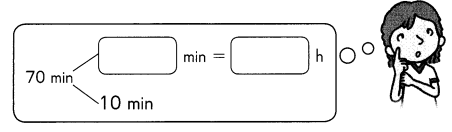70 min = ________ min + ________ min
= ________ h ________ min
There are ___________ hour ___________ minutes in 70 minutes.
Explanation: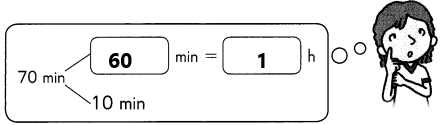We already know, 1 hour=60 minutes.
70 min=60 min+10 min
70 min=1 hour 10 min
There is 1 hour 10 minutes in 70 minutes.

Express the time in hours and minutes.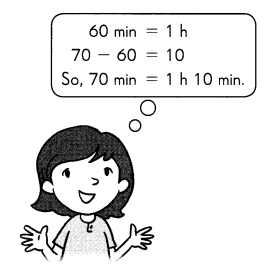Question 20.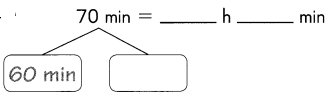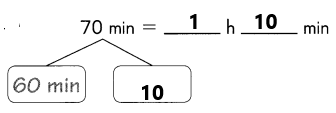We already know, 1 hour=60 minute’s.
70 min=60 min+10 min
70 min=1 hour 10 min
There is 1 hour 10 minutes in 70 minutes.

Question 21.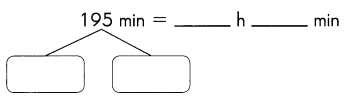Explanation:
195 can be divided into 180 and 15.
If we add 180 and 15 then we get:180+15=195.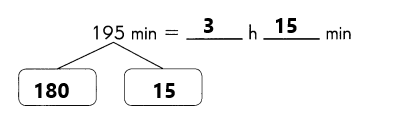To convert minutes into hours, we have to divide the number of minutes by 60.
we already know, 1 hour=60 minute’s.
We need to convert 180 minutes into hours. Assume it as ‘X’ hours.
Now calculate hours. This can be done as:
180 minutes= X hours.
1 hour = 60 minute’s.
Divide 180 and 60 to get the hours.
X=180/60
X=3 hours.
Therefore, 180 minutes=3 hours.
Finally, 195 minutes=3 hours 15 minutes.

Question 22.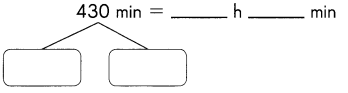Explanation: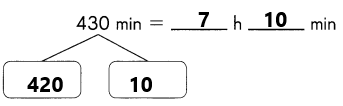430 can be divided into 420 and 10.
If we add 420 and 10 then we get:420+10=430
To convert minutes into hours, we have to divide the number of minutes by 60.
we already know, 1 hour=60 minute’s.
We need to convert 420 minutes into hours. Assume it as ‘X’ hours.
Now calculate hours. This can be done as:
420 minutes= X hours.
1 hour = 60 minute’s.
Divide 420 and 60 to get the hours.
X=420/60
X=7 hours.
Therefore, 420 minutes=7 hours.
Moreover, the remaining 10 minutes will stay the same as the minutes.
Finally, 420 minutes = 7 hours 10 minutes.

Question 23.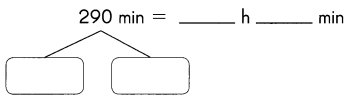Explanation:
290 can be written as 240 and 50.
If we add 240 and 50 then we get 240+50=290.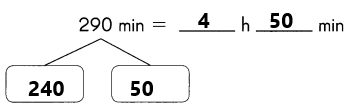To convert minutes into hours, we have to divide the number of minutes by 60.
we already know, 1 hour=60 minute’s.
We need to convert 240 minutes into hours. Assume it as ‘X’ hours.
Now calculate hours. This can be done as:
240 minutes= X hours.
1 hour = 60 minute’s.
Divide 240 and 60 to get the hours.
X=240/60
X=4 hours.
Therefore, 240 minutes=4 hours.
Moreover, the remaining 50 minutes will stay the same as the minutes.
Finally, 290 minutes=4 hours 50 minutes.

Question 24.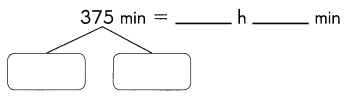Explanation:
375 can be written as 360 and 15.
If we add 360 and 15 then we get 360+15=375.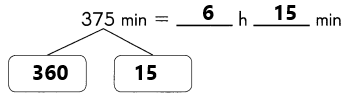To convert minutes into hours, we have to divide the number of minutes by 60.
we already know, 1 hour=60 minute’s.
We need to convert 360 minutes into hours. Assume it as ‘X’ hours.
Now calculate hours. This can be done as:
360 minutes= X hours.
1 hour = 60 minute’s.
Divide 360 and 60 to get the hours.
X=360/60
X=6 hours.
Therefore, 360 minutes=6 hours.
Moreover, the remaining 15 minutes will stay the same as the minutes.
Finally, 375 minutes=6 hours 15 minutes.

Question 25.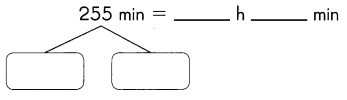255 can be written as 240 and 15.
If we add 240 and 15 then we get 240+15=255.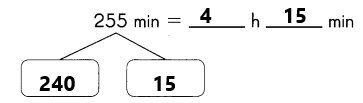To convert minutes into hours, we have to divide the number of minutes by 60.
we already know, 1 hour=60 minute’s.
We need to convert 240 minutes into hours. Assume it as ‘X’ hours.
Now calculate hours. This can be done as:
240 minutes= X hours.
1 hour = 60 minute’s.
Divide 240 and 60 to get the hours.
X=240/60
X=4 hours.
Therefore, 240 minutes=4 hours.
Moreover, the remaining 15 minutes will stay the same as the minutes.
Finally, 255 minutes=4 hours 15 minutes.